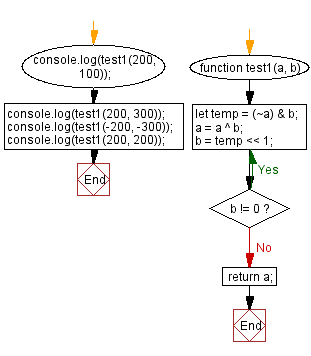# JavaScript: Subtract without arithmetic operators

## JavaScript Math: Exercise-76 with Solution

Write a JavaScript program to subtract one integer from another, without using arithmetic operators such as -, %, /, +, etc.

Test Data:
(200, 100) -> 100
(200, 300) -> -100
(-200, -300) -> 100
(200, 200) -> 0

Sample Solution:

HTML Code:

``````<!DOCTYPE html>
<html>
<meta charset="utf-8">
<title>JavaScript program to Subtract without arithmetic operators</title>
<body>

</body>
</html>
```
```

JavaScript Code:

``````function test1(a, b)
{
do
{
let temp = (~a) & b;
a = a ^ b;
b = temp << 1;
}while (b != 0)
return a;
}

console.log(test1(200, 100));
console.log(test1(200, 300));
console.log(test1(-200, -300));
console.log(test1(200, 200));
```
```

Sample Output:

```100
-100
100
0
```

Flowchart:Live Demo:

See the Pen javascript-math-exercise-76 by w3resource (@w3resource) on CodePen.

Improve this sample solution and post your code through Disqus

What is the difficulty level of this exercise?

Test your Programming skills with w3resource's quiz.

﻿